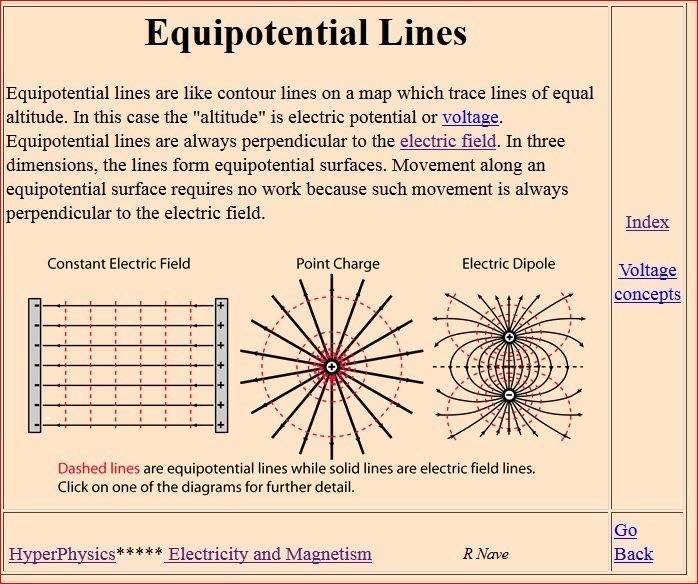# Circuitry Confusion

I have a number of questions on how circuits really function - my class just found itself looking at circuit diagrams and voltages without any explanation of the basics of how circuits actually work.

1) How does the concept of electric potential difference between two points, like with a point charge, relate to circuits? How is it possible to have a "potential difference" between one point of a circuit and another, when there is no field between them? I have the same confusion with capacitors - how is there a potential difference (voltage) between the plates of the capacitor?

2) Similarly, how do things like batteries have voltages? Why does attaching a battery to the circuit, having a voltage (applying a potential difference), make everything flow through the circuit to cause it to function?

An analogy is the idea that you can have a water tank in your roof, and have pipes that transport the water to 'Earth' level.
The flow of water can do useful things while moving down, and on a grand scale a hydroelectric dam can do even more useful things.
Energy can be converted into different forms, potential energy is one of them.
Analogies are never perfect, but it's a way to look at it.

Dale
Mentor
2020 Award
I have a number of questions on how circuits really function - my class just found itself looking at circuit diagrams and voltages without any explanation of the basics of how circuits actually work.
It sounds like your professor had the right approach. There are three different theories of electromagnetism. The most general is quantum electrodynamics. It covers all known EM behavior. One useful simplification is Maxwells equations, which applies in the classical limit. A further simplification is circuit theory, which applies in the small circuit and lumped element limit.

There is no need to bring QED into a circuits class. I agree with keeping the material separate.

1) How does the concept of electric potential difference between two points, like with a point charge, relate to circuits?
In the appropriate limit they are the same thing, but the important thing for circuits is KVL and KCL rather than Coulomb's law.

How is it possible to have a "potential difference" between one point of a circuit and another, when there is no field between them?
If you solve Maxwells equations you will find that there is a field, but all of the added complexity is unnecessary.

I have the same confusion with capacitors - how is there a potential difference (voltage) between the plates of the capacitor?
In circuit theory the important thing is how the voltage is related to the current at the terminals. What goes on inside the terminals is a topic for a class on Maxwells equations or QED.

I have a number of questions on how circuits really function - my class just found itself looking at circuit diagrams and voltages without any explanation of the basics of how circuits actually work.

1) How does the concept of electric potential difference between two points, like with a point charge, relate to circuits? How is it possible to have a "potential difference" between one point of a circuit and another, when there is no field between them? I have the same confusion with capacitors - how is there a potential difference (voltage) between the plates of the capacitor?

2) Similarly, how do things like batteries have voltages? Why does attaching a battery to the circuit, having a voltage (applying a potential difference), make everything flow through the circuit to cause it to function?

To add on to what Dale said--the idea that we can split a circuit up into a bunch of discrete elements connected by wires with zero resistance, where a potential difference immediately gets from point A to B, etc., is just an approximation. It's a pretty good approximation for lower frequencies, but you'll find that at a certain point (namely, when the length of the wire ##\ell## and the wavelength of the signal ##\lambda## are such that ##\ell/\lambda## is sufficiently large) the approximation breaks down, and you'll really have to use Maxwell's equations.

The key concept to understand from a circuit analysis point of view is that an ideal battery in a circuit will always maintain the same potential difference between its terminals.

•Dale
jim hardy
Gold Member
Dearly Missed
How is it possible to have a "potential difference" between one point of a circuit and another, when there is no field between them?

hmmm interesting questions you pose.

How did you arrive at "there is no field between them" ?

Does a simple flashlight battery not have a field, admittedly feeble, between its ends?
Has teacher introduced the concept of "nodes" ?

Try looking at a circuit diagram and think about the main difference between a wire and a circuit element like a resistor.
It helps to imagine yourself in Flatland so there's only two dimensions to handle....
and start with DC , AC comes after we've trained our mind a bit
I think of wire as an equipotential surface
which it almost is,
so there's maybe a field perpendicular to the wire
with its E line originating on a free charge in the wire
and terminating on a free charge in a different wire on other side of the circuit
separated by circuit elements like resistors or capacitors or motors....

http://hyperphysics.phy-astr.gsu.edu/hbase/electric/equipot.htmli have no idea if any of this is a useful direction of thought for you to pursue....

Has teacher taught you to immerse yourself in the circuit and (imagine yourself) walk(ing) around it, tabulating voltages for Kirchoff's law ?

That's how i was taught back when slide rules roamed the earth.
But i'm an analog dinosaur.
You're probably versed in Vector Calculus and Matrices and in need of higher level discourse,
i just thought maybe i'd try priming the well.

old jim

•dlgoff